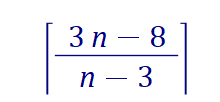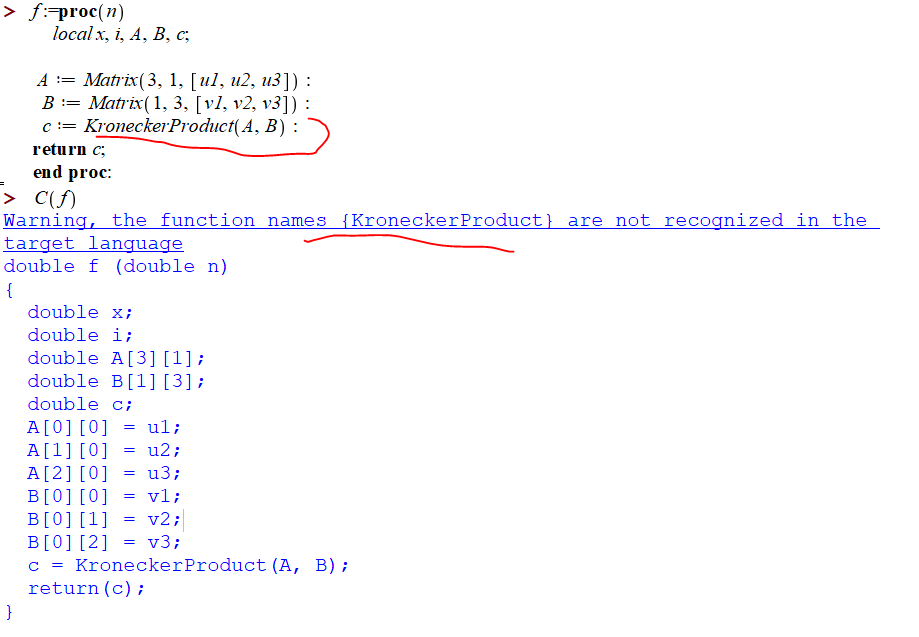## Maple 2022 and Maple 2021 typing slow (Solved!)...

If I type seq(i,i=1..1000) <enter> I get a large output.

Then when I type

for i from 1 to 5 do
i;
end do;

It takes Maple 8 seconds to complete the job.  Is this normal when using Windows 7?

## Which of the following is closest to the total dis...## Maple Flow - Problems printing documents...

Hello,

I am having problems printing Maple Flow documents. I have set up both a header and a footer. In the Print Preview mode, everything is fine. When I try to print to a PDF file, the header loses its format and the footer does not print at all. Printing to a printer looks the same. What settings must be selected so that the printout can be passed on as a well-formatted document?## Older maple manuals. Link on Maplesoft no longer ...

Did Maple just end the links to older maple manuals?

I can't locate the link to find older maple manuals on the maplesoft website.  Did it get moved?  I can't find it.  Does anyone have a link?

## Getting coordonates of points from an image...

Hello Everybody

My name is Emmanuel and i live in Paris, France. I work in insurance and i am an actuary.

I am a new maple user because i wanted to have some fun redoing some mathematics. (I studied mathematic 30 years ago).

I would like to develop an animation with epicyles to draw something.

(there is a famous one on youtube with homer simpson)

My idea is to embed an image with a simple drawing, get the coordonate from the points and apply a complex fourier transfor to get the parameters and make an animation.

I found the way to make the animation, to emben the image. .But i cant find how to get a maxtrix or a vector (i dont know what is the best ) of the coordinate of the point of the drawing. Does any of you have an idea to do it ?

Thanks a lot

## How to "catch" warning messages from a procedure....

with(Optimization):
expr_optimize := fSW__Ratio_Tol[XU5]*f_ratio__SpreadSpectrum[XU5]*(R34^2*k1__RT[XU5] + R34*k2__RT[XU5] + k3__RT[XU5])/R34^2;
seqUnknownRanges := R34 = 5.640*10^4*Unit('Omega') .. 6.160*10^4*Unit('Omega'), k1__RT[XU5] = 4.054*10^4*Unit(1/('s')) .. 4.054*10^4*Unit(1/('s')), k2__RT[XU5] = 1.593*10^11*Unit(1/('F')) .. 1.593*10^11*Unit(1/('F')), k3__RT[XU5] = -2.645*10^15*Unit('m'^4*'kg'^2/('s'^7*'A'^4)) .. -2.645*10^15*Unit('m'^4*'kg'^2/('s'^7*'A'^4)), fSW__Ratio_Tol[XU5] = 0.950 .. 1.050, f_ratio__SpreadSpectrum[XU5] = 1 .. 1.250;
NLPSolve(expr_optimize, seqUnknownRanges, 'useunits', 'method' = 'modifiednewton', 'optimalitytolerance' = 0.101);

NLPSolve returns this message "Warning, convergence is not assured; examine Hessian values, or consider raising tolerance"

How can I capture this message in a procedure and take appropriate action?

## Is there a way to see the Maple implementation of ...

Is there a way to see the Maple implementation of Runge Kutta 4th order method in dsolve?

By searching I found I can print the implementation of a procedure as follows

interface(verboseproc = 2)

print(dsolve)

But I'd like specifically to see the code for the method RK45.

## A simplification problem involving ceil...

It is easy to simplify the following expression (to 4), but maple's `ceil` function does not seem to be interested in simplifying it.where n is greater than or equal to 4.

```simplify(ceil((3*n-8)/(n-3))) assuming n>=4, n::positive # As-is output
```

We have to rewrite "(3*n-8)/(n-3)" in this form "3 + 1/(n - 3)" to recognize.

`simplify(ceil(3 + 1/(n - 3))) assuming  n>=4, n::positive`

4

• My first question is: How to transform (3*n-8)/(n-3) into 3 + 1/(n - 3) by maple?
• My second question: Can we see the steps of execution of the simplification involving  `ceil`)?

## CodeGeneration for LinearAlgebra problems...

I am trying to generate c code for a LinearAlgebra test problem using Maple:

But the output cannot be used due to the warning:

Warning, the function names {KroneckerProduct} are not recognized in the target language

The proposed code looks like:## How to resolve of this ODE System Error...

How to resolve of this ODE System Error, the proble is "Error, (in dsolve/numeric/process_input) input system must be an ODE system, got independent variables {t, [S, E, In, R]}

## Is it possible to have a combobox with two columns...

I need a combobox with two columns but I don't think it is possible.  I want to display a list of acids with their names and dissociation constants.

## Store values between sessions including nested tab...

Don't know if this is a bug or just another proof of my incompetence, but I am struggling in storing values between sessions using DataTable components.

The problem seems to me to be related to nested tables, somehow.

I've made a little example showing the problem.

Quickly explained, you should do 2 runs to see the problem. The first run creates a matrix and a table, and stores the values in a DataTable cell. You shouldn't see any problems there.

Save the document and close it.

Then open the file once again, but just run the 2 commands in chapter 4. You should then see an error message.

My goal is to be able to save a nested variables with its values in a Datatable between sessions. The main variable and many of them below are tables, the last in the branch often string values or numbers with units.

StoreBetweenSessions.mw

## How to make subs only works in exponential?...

How should I change w1 to w2 only in exponential? For example, let

`x := omega1*exp(omega1*t1*I) + omega1/exp(omega1*t1*I)`

expect result:

`x := omega1*exp(omega2*t1*I) + omega1/exp(omega2*t1*I)`

## how to define proc that returns expression sequenc...

I want to make proc that returns expression sequence of two numbers, say. A,B

This will be the normal return, but if the proc encounters an error, it should return FAIL. But this is not possible, because if I type A,B:=foo(0) and foo() happenes to return FAIL then Maple will complain

Error, mismatched multiple assignment of 2 variables on the left side and 1 value on the right side

The three workarounds I see are these

Method 1

I could wrap foo() with try/catch and have foo throw an exception when it encouters an error instead of returning FAIL, but I do not like this. This will look like

```restart;
interface(warnlevel=4);
kernelopts('assertlevel'=2):
foo:=proc(n::integer)::integer,integer;
if n=0 then
error ("encountered error");
else
return 1,2;
fi;
end proc;
```

And now the caller will do

```try
A,B:=foo(0);
catch:
print("encountered error");
end try;```

method 2

Another option is to have foo() return ONE value back. In the case of an error it will be FAIL, and in the case of normal behavior, it will be a LIST of the two items, which then I have to extract.  Like this

```interface(warnlevel=4);
kernelopts('assertlevel'=2):
foo:=proc(n::integer)::Or(list(integer),identical(FAIL));
if n=0 then
return FAIL;
else
return [1,2];
fi;
end proc;
```

And now the caller will do this

```L:=foo(1);
if L<>FAIL then
A:=L;
B:=L;
else
print("failed call");
fi;```

But this is not as easy as just calling foo() as   A,B:=foo() but I can't do this due to the mismatch problem.

Another method, commonly used in C programming is to have the function return status an additional output and this will indicate if the call was success or not. so the caller has to remember to always check for this before using the returned values. These are handled by errno, perror(), strerror(). But for now, lets make the function return the status.

So caller will first check if status is success first and if so, then it will read the actual returned values that follow it. Like this (status is true/false)

method 3

```interface(warnlevel=4);
kernelopts('assertlevel'=2):
foo:=proc(n::integer)::truefalse,integer,integer;
if n=0 then
return false,0,0;
else
return true,1,2;
fi;
end proc;
```

And the caller will do

```status,A,B:=foo(1);
if status then
print("call was success, A and B are ",A,B);
else
print("call failed");
fi;
```

So the return values will always have status as first argument that the caller has to check before reading the rest of returned values.

From the above three options which you think is best?

Anyone can suggest alternatives to the above methods?